# 实验一 Python 基本操作 主要实验内容 print 、input 语句、pip 安装第三方库以及标准库的使用 1. print 语句

``````print('''
@@@@@@@@@@@
@@@@  @@@@
@@@ @ @@@
@@ @@@ @@
@@@ @ @@@
@@@@  @@@@
@@@@@@@@@@@
'''
)
print('''
++++++++++++
+     +
+++++++++++++
''')
print('''

''')``````

2. input 语句
（1）编程程序，输入直角三角形的两个直角边的长度 a、b，求斜边c 的长度。

``````import math
a=float(input('输入直角三角形其中一个直角边'))
b=float(input('输入直角三角形其中一个直角边'))
c=math.sqrt(a*a+b*b)
print(c)
``````

（2）编程程序，用于实现两个数的交换。

``````a=eval(input('请输入a'))
b=eval(input('请输入b'))
a,b=b,a
print('a:',a)
print('b:',b)``````

``````a=eval(input('请输入成绩'))
b=eval(input('请输入成绩'))
c=eval(input('请输入成绩'))
he=a+b+c
average=he/3
print(average)``````

（4）编程程序，根据输入的三角形的三边长度，输出三角形的面积
（提示：利用海伦公式、引入 math 库(import math)，并调用 math.sqrt()函数

``````import math
a=eval(input('输入三角形的边'))
b=eval(input('输入三角形的边'))
c=eval(input('输入三角形的边'))
p=(a+b+c)/2
S=math.sqrt(p*(p-a)*(p-b)*(p-c))
print(S)``````

1、用户输入一个三位自然数，计算并输出其百位、十位和个位上的数字。

``````a=eval(input('输入一个三位自然数'))
b=a%10
c=int(a/10)
d=c%10
e=int(a/100)
print(b,d,e)``````

2、编写程序， 输入任意大的自然数， 输出各位数字之和

``````a=eval(input('输入一个三位自然数'))
he=0
while a>0:
b=a%10
he+=b
a=int(a/10)
print(he)
``````

3、编写程序，输入两个集合 setA 和 setB，分别输出它们的交集、并集和差集 setA-setB。

``````setA={1,23,34,45}
setB={23,12,35}
a=setA & setB
b=setA | setB
c=setA-setB
print(a,b,c)``````

4， 编写程序， 输入一个自然数， 输出它的二进制、 八进制、 十六进制表示形式。提示：ppt 中关于数值类型中相应的公式

``````a=eval(input('输入一个自然数'))
print(bin(a))
print(oct(a))
print(hex(a))``````

5、如下图所示，完成以下两个题目``````a=eval(input('请输入本金'))
b=eval(input('请输入年利率'))
c=eval(input('请输入年数'))
d=a
x=1
while x<=c:
d=d+(b/100)*x*a
x+=1
print('本金利率和为:{:.2f}'.format(d))``````

6 .编写程序,输入球的半径,计算球的表面积和体积(结果保留两位小

(1)球的表面积的计算公式为 4*π*r2,球的体积的计算公式为 4/3*π*r3
(2)用 户 可以 使 用 “ print(str.format(" 球 的 表面 积 为 :{0:2.2f},体 积为:{1:2.2f}",area, volume))”的语句形式输出程序运行效果。

``````r=eval(input('输入球的半径'))
S=4*3.14*r*r
V=4/3*(3.14*r**3)
print('球的表面积:{:.2f},球的体积:{:.2f}'.format(S,V))``````

7. 编写程序, 根据输入本金 b、年利率 r 和年数 n 计算最终收v,v=b(1+r)";然后编写测试代码,提示输入本金、年利率和年数,显示最终收益(保留两位小数)。

``````b=eval(input('请输入本金'))
r=eval(input('请输入年利率'))
n=eval(input('请输入年数'))
v=b*(1+r)**n
print('最终收益:{:.2f}'.format(v))``````

8.编写程序,求解一元二次方程 x2-10x+16=0,运行效果参见下图。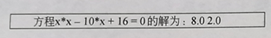``````import math
a=1
b=-10
c=16
d=b*b-4*a*c
x=(-b+math.sqrt(d))/2*a
x2=(-b-math.sqrt(d))/2*a
print('方程x*x-10*x+16=0的解为:{},{}'.format(x,x2))``````

9.编写程序,提示输入姓名和出生年份,输出姓名和年龄,运行效果参见

(2)用户可以使用“ print("您好!{0}。您{1}岁。". format( SName,age)”的语句形式输出程序运行效果.

``````import datetime
Sname=input('输入姓名')
Snian=eval(input('输入出生年份'))
now=datetime.date.today().year
age=int(now-Snian)
print('您好!{0},您{1}岁'.format(Sname,age))
``````

10. 输入直角三角形的两个直角边，求三角形的周长和面积，以及两个锐角的角度。结果均保留一位小数。效果如下图。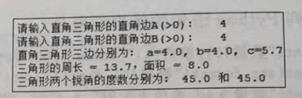提示：
(1)math.asin()函数返回正弦值为指定数字的弧度;math.acos()函数返回余弦值为指定数字的弧度。
(2)将弧度转换为角度的公式为角度=弧度*180/π
(3)可以使用“ round(asin(sinA)*180/pi,0)”的语句形式求锐角 A 的度数
(4) 可 以 使 用 “print(str. format(" 三 角 形 的 周 长 ={0:1.1f}, 面 积={1:1.1f}",p,area)”的语句形式按题目要求输出三角形的周长和面积。

``````import math
a=eval(input('请输入直角边A'))
b=eval(input('请输入直角边B'))
c=math.sqrt(a*a+b*b)
print('直角三角形三条边分别为:{:.1f},{:.1f},{:.1f}'.format(a,b,c))
C=a+b+c
S=(a+b)/2
print('三角形的周长:{:.1f},面积:{:.1f}'.format(C,S))
d=math.asin(a/c)
e=math.asin(b/c)
d1=d*180/3.14
e2=e*180/3.14
print('三角形两个锐角的度数分别为:{:.1f},{:.1f}'.format(d1,e2))``````

# 实验二 程序流程控制 1. 编写程序计算 1+3+5+7…+99 之和。

``````a=1
he=0
while a<=99:
he=he+a
a=a+2
print(he)    ``````

2. 编写程序,计算 2+4+6+8…+100 之和.

``````a=2
he=0
while a<=100:
he=he+a
a=a+2
print(he)``````

3. 编写程序,使用不同的实现方法输出 2000~3000 的所有闰年,运行效果如下图所示。提示：闰年包括普通闰年和世纪闰年，修改 print 函数中的‘end’选项。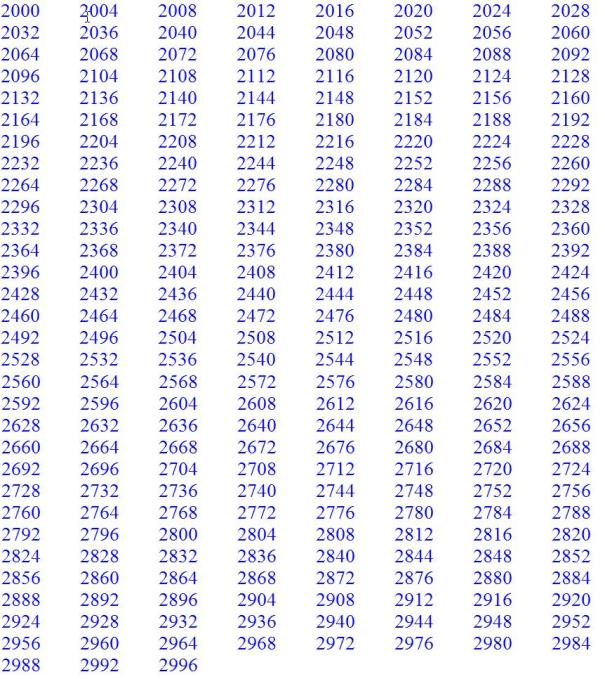``````num=0
for i in range(2000,3001):
if (i%4==0 and  i%100!=0) or i%400==0:
num+=1
if num%8==0:
print(i,end='\n')
else:
print(i,end='\t')
else:
i=i+1``````

4. 编写程序,计算 Sn=1-3+5-7+9-11+…

``````x=1
sn=0
b=eval(input('请输入任意整数'))
for i in range(1,b+1,2):
sn=sn+i*x
x=-x
print(sn)    ``````

5. 编写程序,计算 Sn=1+1/2+1/3+…。

``````sn=0
b=eval(input('请输入任意整数'))
for i in range(1,b+1):
x=1/i
sn=sn+x
print(sn)``````

6. （只要实现四种方式中的一种即可）编写程序,打印九九乘法表。要求输出九九乘法表的各种显示效果(上三角、下三角、矩形块等方式)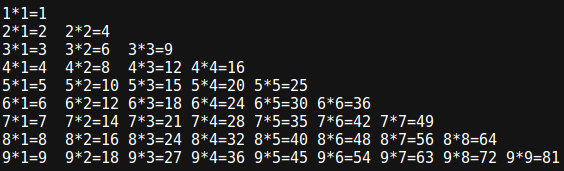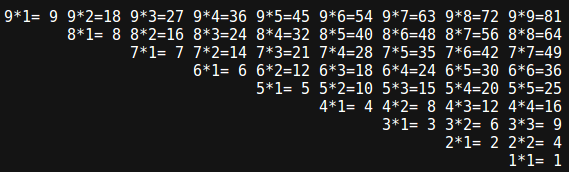``````for i in range(1,10):
for j in range(1,i+1):
c=i*j
print('{}*{}={}'.format(i,j,c),end='\t')
print(end='\n')     ``````

7. 编写程序输入三角形的 3 条边,先判断是否可以构成三角形,如果可以,则进一步求三角形的周和面积,否则报错“无法构成三角形!”。其运行效果如图下图所示(结果均保留位小数)。(2)已知三角形的 3 条边,则三角形的面积= h h a h b h c       ( ) ( ) ( )，其中 h 为三角形周长的一半。
(3)其中根号需要导入 math 库并调用 sqrt 函数

``````import math
a=eval(input('请输入三角形的边长a:'))
b=eval(input('请输入三角形的边长b:'))
c=eval(input('请输入三角形的边长c:'))
if a+b>c:
print('三角形三边分别为:a={:.1f},b={:.1f},c={:.1f}'.format(a,b,c))
h=(a+b+c)/2
S=math.sqrt(h*(h-a)*(h-b)*(h-c))
print('三角形的面积:{}'.format(S))
else:
print('构不成三角形')``````

8. 编写程序,输入 x,根据如下公式计算分段函数 y 的值。请分别利用单分支语句双分支结构以及条件运算语句等方法实现。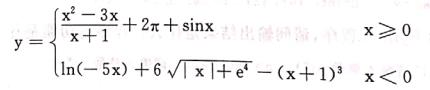``````import math
x=eval(input('请输入x:'))
if x>=0:
y=(x*x-3*x)/(x+1)+2*math.pi+math.sin(x)
else:
y=math.log(-5*x)+6*math.sqrt((-x)+math.e**4)
print(y)
``````

9. 编写程序,输入一元二次方程的 3 个系数 a、b 和 c,求 ax2+bx+c=0 方程的解。结果如下图所示

(1)方程 ax2+bx+c=0 的解有以下几种情况。
①a=0 and b=0,无解。
②a=0andb!=0,有一个实根
③b2-4ac=0,有两个相等实根
○4b2-4ac>0,有两个不等实根
⑤b2-4ac<0,有两个共轭复根
(2)可以利用“ print(str. format("此方程有两个不等实根:{0}+{1}和{0}-{1}",realPart, imag Part,)”的语句形式输出方程的两个共轭复根。

``````import math
a=eval(input('请输入a:'))
b=eval(input('请输入b:'))
c=eval(input('请输入c:'))
t=b*b-4*a*c
if a==0 and b==0 and c!=0:
print('无解')
elif a==0 and b!=0:
print('有一个实根')
elif t==0:
s=(-b+math.sqrt(t))/2*a
s1=(-b-math.sqrt(t))/2*a
print('有两个相等的实根:{:.1f},{:.1f}'.format(s,s1))
elif t>0:
s=(-b+math.sqrt(t))/2*a
s1=(-b-math.sqrt(t))/2*a
print('有两个不相等的实根:{:.1f},{:.1f}'.format(s,s1))
elif t<0:
s2=-b/(2*a)
s3=math.sqrt(-t)/2*a
print('有两个共轭复根:{0}+{1}i和{0}-{1}i'.format(s2,s3))``````

10. 编写程序,输入整数 n(n≥0),分别利用 for 循环和 while 循环求 n!。

(1) n!=n×(n-1)×(n-2)×…×2×1。例如 5!=5×4×3×2×1=120,特别地,0!=1
(2) 一般情况下,累乘的初值为 1,累加的初值为 0。
(3) 如果输入的是负整数,则继续提示输入非负整数,直到 n≥0。

``````n=int(input('请输入一个数'))
s=1
if n==0:
print('1')
for i in range(1,n+1):
s*=i
i+=1
print(s)
n=int(input('请输入一个数'))
s=i=1
if n==0:
print('1')
while i <=n:
s *=i
i +=1
print(s)  ``````

11.编写程序,产生两个 0~100(包含 0 和 100)的随机整数 a 和 b,求这两个整数的最大公约数和最小公倍数。

(2)利用“辗转相除法”求最大公约数,具体算法如下。
①对于已知的两个正整数 m、n,使得 m>n
②m 除以 n 得余数 r
③若 r≠0,则令 m+n,n←r,继续相除得到新的余数 r。若仍然 r≠0,则重复此过程,直到 r=0 为止。最后的 m 就是最大公约数。(3)求得了最大公约数,最小公倍数就是已知的两个正整数之积除以最

``````import random
a=random.randint(1,100)
b=random.randint(1,100)
print(a,b)
if a>b:
n1=a
n2=b
while (n2!=0):
c=n1%n2
n1=n2
n2=c
print("最大公约数",n1)
print("最小公倍数",a*b/n1)  ``````

# 实验三 Python 组合数据类型 1. 创建列表以列表 list1 = ["lift", "is", "short"]和 list2 = ["You", "need", "python"]，并完成以下任务: (1)输出 listl 中的第一个元素 lift 及其索引(下标)。 (2)在 short 后面增加一个!

``````list1 = ["lift","is","short"]
list2 = ["You","need","python"]
print(list1)
print(list1.index('lift'))
list1.append("!")
print(list1)``````

2. 编写程序,将列表 s=[9,7,8,3,2,1,5,6]中的偶数变成它的平方,奇数保持不变。其运行效果如下图所示。

 变换前,s=[9,7,8,3,2,1,5,6] 变换后,s=[9,7,64,3,1,5,36]
``````s=[9,7,8,3,2,1,5,6]
print('变换前',s)
for i in range(len(s)):
if s[i]%2==0:
s[i]=s[i]**2
else:
s[i]=s[i]
print('变换后',s)        ``````

3. 分别使用 if 和 while 语句删除将列表 x = [1,2,1,2,1,1,1]中的元素“1”全部删除。

``````x = [1,2,1,2,1,1,1]
fre=x.count(1)
for i in range(fre):
x.remove(1)
print(x)
#方法二
y=x[:]
for j in y:
if j==1:
x.remove(1)
print(x)
#方法三
x1=[]
for i in range(len(x)):
if x[i]==2:
x1.append('2')
print(x1)       ``````

5. (选做)统计所输入字符串中单词的个数,单词之间用空格分隔。其运行效果如下图所示。

 请输入字符串: The qu1ck brown fox jumps over the lazy dog. 其中的单词总数有:9
``````str1='The qu1ck brown fox jumps over the lazy dog'
list1=str1.split(' ')
print('其中的单词总数为',len(list1))``````

6. 编写程序,输入字符串,将其每个字符的 ASCI 码形成列表并输出,运行效果如下图所示。

(2)使用 s. append(x)方法将对象 x 追加到列表 s 的尾部。``````s=input('请输入一个字符串')
s1=[]
for i in range(len(s)):
c=ord(s[i])
s1.append(c)
print(s1)

``````

7. (选做)创建列表,内容为 a-z、A~Z 和 0-9,从中随机抽出 6 个字符作为验证码。提示:使用 ASCII 值和 chr 函数（chr()主要用来表示 ascii码对应的字符他的输入时数字，可以用十进制，也可以用十六进制）生成大小写字母或 string 库。

``````import random
lists1=[]
list1=[chr(i) for i in range(97,123)]
lists1.extend(list1)
list2=[chr(i) for i in range(65,91)]
lists1.extend(list2)
list3=list(range(10))
lists1.extend(list3)
code=[]
l=len(lists1)
for i in range(6):
r=random.randint(0,l-1)
code.append(lists1[r])
print(code)``````

8. 使用列表实现筛选法求素数。提示：素数判断方法：用一个数分别去除 2 到 sqrt(这个数)，如果能被整除，则表明此数不是素数，反之是素数。

``````import math
list1=[]
a=eval(input("请输入一个数"))
x=math.sqrt(a)+1
if a==3:
print('是素数')
list1.append(a)
print(list1)
if a!=3:
for i in range(2,int(x)):
if a%i==0:
print('不是素数')
else:
print('是素数')
list1.append(a)
print(list1)``````

9. 编写程序,求列表 s=[9,7,8,3,2,1,55,6]中的元素个数、最大值、最小值,以及元素之和、平均值。请思考有哪几种实现方法?

``````s=[9,7,8,3,2,1,55,6]
num=0
for i in s:
num+=1
s1=max(s)
s2=min(s)
s3=sum(s)
s4=s3/num
print(s1,s2,s3,s4,num)    ``````

10.创建一个空字典 student,录入学生姓名和成绩,并一一对应,当所有学生的信息录入完之后,输入 1 退出。需要注意的是(学生成绩范围为 0~150)超出范围重新输入。提示：使用 dict 函数和 zip 函数

``````student={}
alist=[]
blist=[]
prime=0
while prime==0:
a=input('输入学生姓名')
alist.append(a)
b=eval(input('输入学生成绩'))
if b>0 and b<150:
blist.append(b)
else:
print('重新输入')
prime=int(input('是否需要继续，是请输入0，否输入1'))
student=dict(zip(alist,blist))
print(student)    ``````

11.自己编写一个长度至少五 5 的字典，其中姓名为键和性别为值；请编写一个程序,删除性别为男的员工信息。

``````a={'aa':'nan','bb':'nv','cc':'nan','dd':'nan','ee':'nv'}
a1=a.copy()
for key in a:
if a[key]=='nan':
del a1[key]
print(a1)
#方法二
a={'aa':'nan','bb':'nv','cc':'nan','dd':'nan','ee':'nv'}
a1={}
for key in a:
if a[key]=='nv':
a1[key]=a[key]
print(a1)    ``````

12.（选做）请编写一个程序,使用字典存储学生信息,学生信息包括学号和姓名,请根据学生学号从小到大输出学生的信息。提示：使用sorted 函数并指定其对应的参数 key 的值。

``````student={}
alist=[]
blist=[]
prime=0
while prime==0:
a=input('输入学生姓名')
alist.append(a)
b=eval(input('输入学生成绩'))
if b>0 and b<150:
blist.append(b)
else:
print('重新输入')
prime=int(input('是否需要继续，是请输入0，否输入1'))
student=dict(zip(alist,blist))
student=sorted(student.items(),key=lambda x:x)
print(student) ``````

13.（选做）请编写一个程序,用于统计字符串（“asjhsdjkgavsdjasdbj”）中每个字母的出现次数(字母忽略大小写,a 和 A 看是作一个字母)，统计出的结果,请按照[‘a’:3,’b’:2]的格式输出。提示：可以使用 chr函数将字母变为整数，然后利用整数值的大小进行判定。

``````n=97
alist=[]
blist=*26
for i in range(26):
a=chr(i+n)
alist.append(a)
string1=input('请输入字符串')
for i in range(26):
for j in range(len(string1)):
if string1[j]==chr(n+i) or string1[j]==chr(n+i-32):
blist[i]=blist[i]+1
``````

14.使用元组创建一个存储 Python 关键字的对象,并利用 input 函数输入任何一个字符串，来检测输入的单词是否是 Python 的关键字。

``````a=('False','class','from','or','None','contine','global','pass','True','def','if','raise','and','del','import','return','as','elif','in','try','assert')
b=input('请输入要检测的关键字：')
if b in a:
print(b+'是python关键字')
else:
print(b+'不是python关键字') ``````

15.使用集合实现筛选法求素数

``````import math
aset=set()
alist=[]
a=eval(input('输入一个自然数'))
x=math.sqrt(a)+1
if a==3:
if a%(math.sqrt(3))==0:
print('不是素数')
else:
print('是素数')
alist.append(a)
aset=set(alist)
if a!=3:
for i in range(2,int(x)):
if a%i==0:
print(a,'不是素数')
else:
print(a,'是素数')
alist.append(a)
aset=set(alist)
print(aset)

#*********************************************************************
def sushu(num):
prime = True
for i in range(2,num):
if num%i == 0:
print(str('%d' % num)+'不为素数' )
prime = False
break
if prime is True:
print(str('%d' % num)+'为素数')
aset=set()
alist=[]
n=int(input('请输入集合元素的个数：'))
for i in range(n):
a=int(input('请输入元素：'))
alist.append(a)
aset=set(alist)
alist=list(aset)
print('The Judgement:')
for i in range(len(alist)):
sushu(alist[i])``````